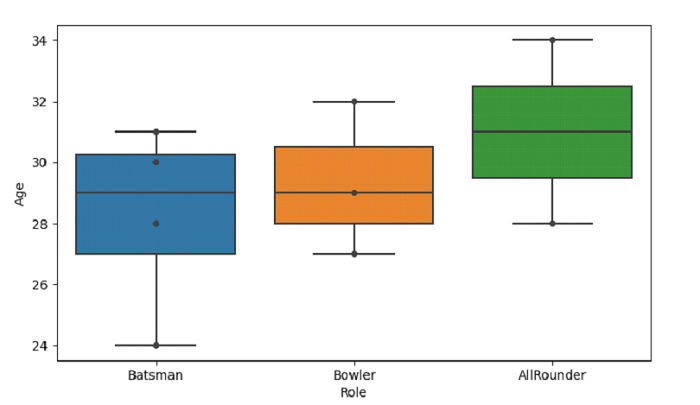# Python Pandas - Draw a boxplot and display the datapoints on top of boxes by plotting Swarm plot with Seaborn

PythonServer Side ProgrammingProgramming

To plot swarm plot on top of box plot, at first, set boxplot() and then the swarmplot() with the same x and y values. Box Plot in Seaborn is used to draw a box plot to show distributions with respect to categories. The seaborn.boxplot() is used for this.

Swarm Plot in Seaborn is used to draw a categorical scatterplot with non-overlapping points. The seaborn.swarmplot() is used for this.

Let’s say the following is our dataset in the form of a CSV file − Cricketers.csv

At first, import the required libraries −

import seaborn as sb
import pandas as pd
import matplotlib.pyplot as plt

Load data from a CSV file into a Pandas DataFrame −

dataFrame = pd.read_csv("C:\\Users\\amit_\\Desktop\\Cricketers.csv")


Plotting swarm plot on top of box plot −

sb.boxplot( x = 'Role',y = 'Age', data = dataFrame )

sb.swarmplot(x="Role", y="Age", data=dataFrame, color=".25")

## Example

Following is the code −

import seaborn as sb
import pandas as pd
import matplotlib.pyplot as plt

# Load data from a CSV file into a Pandas DataFrame:

# plotting box plot
sb.boxplot( x = 'Role',y = 'Age', data = dataFrame )
# plotting swarn plot on top of box plot
sb.swarmplot(x="Role", y="Age", data=dataFrame, color=".25")

# display
plt.show()

## Output

This will produce the following output −